## Dividing Polynomials

##### Example 1

Simplify each of the following division expressions and find the pattern involving the exponents.

1.2.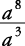1.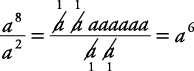2.These examples suggest the following rule: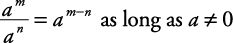##### Example 2

Simplify each of the following:

1.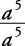2.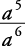3.1.2.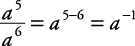, also, so3.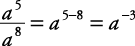, also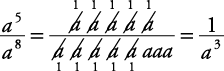, so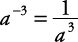These examples suggest the following rule: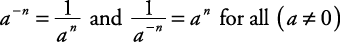Generally, when simplifying expressions, write the final result without the use of negative exponents.

##### Example 3

Simplify each of the following.

1.2.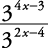1.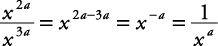2.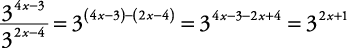To divide a monomial by another monomial, follow this procedure:

1. Divide the numerical coefficients.

2. Divide the variables.

3. Write the results as a quotient.

##### Example 4

Simplify the following expressions by dividing correctly.

1.2.3.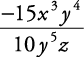1.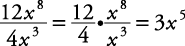2.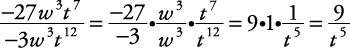3.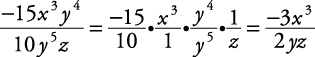To divide a polynomial by a monomial, divide each term of the polynomial by the monomial.

##### Example 5

Simplify the given expression by dividing correctly.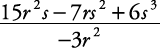To divide a polynomial by a polynomial, a procedure similar to long division in arithmetic is used. The procedure calls for four steps: divide, multiply, subtract, and bring down. This procedure is repeated until there no value is left to bring down.

##### Example 6

Divide x 2 + 3 x 3 – 5 by 4 + x.

First, arrange both polynomials in descending order, filling in a place holder for any missing terms.Now divide 3 x 3 by x and bring this partial quotient to the top as the first part of the answer.Multiply 3 x 2 by the divisor x + 4 and place these in the columns with like terms.Subtract. Remember, to subtract is to add the opposite.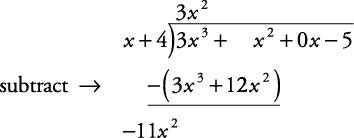Bring down the next term and start the procedure again.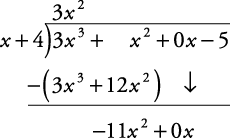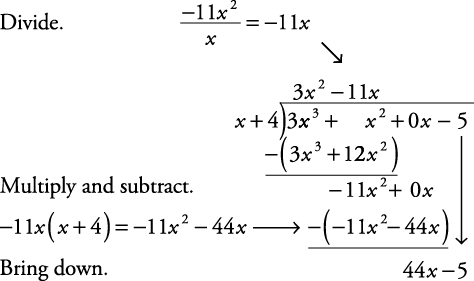Since a value was brought down, start the process over again.At this point, there is no term to bring down. The –181 is the remainder. As in arithmetic, remainders are written over the divisor. So the final answer to the division problem is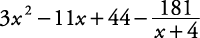##### Example 7

Divide 64 x 3 – 27 by 4 x – 3.

First divide 64 x 3 by 4 x.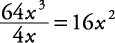Write it above the first term under the long‐division symbol. Then multiply and subtract.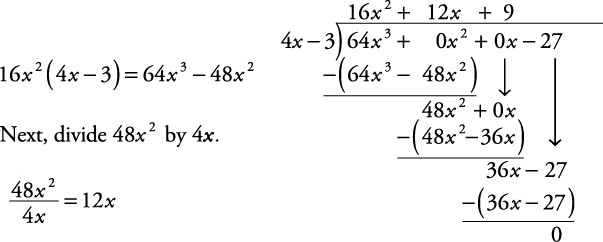Write that above the next term under the long‐division symbol, with a plus sign separating it from the first one. Then multiply and subtract.

Finally, repeat the sequence with the 36 x.The answer is 16 x 2 + 12 x + 9. Notice that there is no remainder. Add: “There is no remainder.”

Back to Top
A18ACD436D5A3997E3DA2573E3FD792A